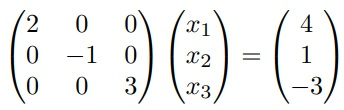# MATLAB code for backward substitution method for solving the linear system

19

## Description

This function uses the backward substitution method for solving the linear system Ax = b, where A is an upper triangular matrix b is a known vector and n is the dimension of the problem.

## Example :```A = diag([2, -1, 3])

A =

2     0      0
0    -1     0
0    0       3

b = [4; 1; 3]

b =

4
1
3

```

The code gives the final result :

```x =

2
-1
1```

## Reviews

There are no reviews yet.

SKU: 1SOUPR0LISY3 Category: